# SCs language

### Common

SCs-code - is a text representation of SC-code. Whole text consist of sentences, that separated by `;;` symbols.

#### Comments

You can use C-style comments in SCs text:

``````// one line comment
fruit -> apple;
/* Multiline
* comment
*/
``````

To make an `sc-link` into specified file you can use special type identifier:

``````"file://<file name>"
``````

• `"file://<file name>"` - is a relative path to a file. According to a file, where it used;
• `"file:///<file_name>"` - is an absolute path to a file.

#### Names

There are some tricks with object names:

• `...` - is an unnamed object;
• `_<object name>` - all object names, that starts with symbol `_` represents a variable type of objects.

Objects identifier visibility. By default all objects with name `x` are visible anywhere. After translating it into memory this object will have a system identifier equal to `x`. So if you use `x` in different scs files, then you designate the same object in them (would be the same element in a knowledge base).

Sometimes you need to designate the same objects in different files, but do not generate a system identifier in memory for it. In this case you should to prefix it name with a `.` symbol. For example: `.x`.

In case, when you need to make a named object just local for an scs file, then you should to use `..` prefix (example: `..x`).

So a rule to build identifier is:

``````[visibility][variable]<identifier>
``````

For example identifier `.._x` localy visible variable identifier.

#### Aliases

You can use alias for any sc-element by using `=` operator. There are some examples:

``````@file_alias = "file://...";;
@link_alias = [];;
@element_alias = element_idtf;;
@edge_alias = (c -> b);;
@alias_to_alias = @other_alias;;
``````

Warning

Aliases visible just in a file scope. You should define alias before usage.

Note

SCs-code is splitted into levels. Each level allows to minimize number of string symbols to represent the same structures.

### SCs level 1

SCs-code level 1 - is a simple representation of SC-code. It represents it with just simple triples. Each triple contains `subject`, `predicate`, `object` that are splitted by `|` symbol. Line `subject | predicate | object;;` is a sentence.

Identifier of `subject`, `predicate`, `object` build with rule:

``````<type>#<identifier>
``````

Where `type` - is an element type specification. It can be one of possible values:

• `sc_node` - equal to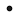;
• `sc_link` - equal to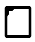;
• `sc_edge_dcommon` - equal to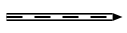;
• `sc_edge_ucommon` - equal to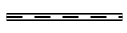;
• `sc_edge_main` - equal to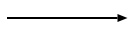;
• `sc_edge_access` - equal to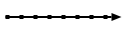.

Examples

SCg construction Equal SCs-level 1 text
```        ```
// append set of apples into fruit set
sc_node#fruit | sc_edge_main#... | sc_node#apple;;
// append set of bananas into fruit set
sc_node#fruit | sc_edge_main#... | sc_node#banana;;
```
```
```        ```
sc_node#apple | sc_edge_dcommon#..e | "file://apple.png";;
/*append edge from nrel_image relation into
edge between apple set and it's image*/
sc_node#nrel_image | sc_edge_main#... | sc_edge_dcommon#..e;;
```
```

SCs-level 1 allows you to represent any kind of SC-code construction. It's a low-level representation and commonly used as a transport format, that is very simple for parsing.

### SCs level 2

This level of SCs-text add two new features:

• using of extended alphabet of edges;
• using of compound identifiers of an edges.

On this level you can make sentences by rule:

``````<element> <edge> <element>;;
``````

Where `<edge>` can be on of values:

SCs SCg equivalent
`<` or `>``<>``<..` or `..>``<=>`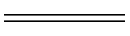`_<=>`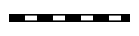`<=` or `=>`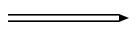`_<=` or `_=>`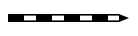`<-` or `->``_<-` or `_->`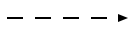`<|-` or `-|>`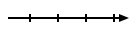`_<|-` or `_-|>`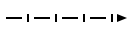`</-` or `-/>`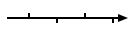`_</-` or `_-/>`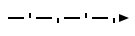`<~` or `~>`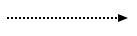`_<~` or `_~>`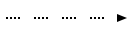`<|~` or `~|>``_<|~` or `_~|>`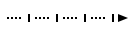`</~` or `~/>`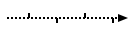`_</~` or `_~/>`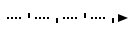SCg construction Equal SCs-level 2 text
```        ```
fruit -> apple;;
// backward direction
banana <- fruit;;
```
```

Compound identifier of an edge builds as a sentence in SCs-level 2, but without `;;` separator and inside brackets `()`: `(<element> <edge> <element>)`. So that allows to simplify usage of an edge as a source or target of another one.

Examples

SCg construction Equal SCs-level 2 text
```        ```
nrel_image -> (fruit => "file://apple.png");;
```
```
```        ```
d -> (c -> (a -> b));;
```
```
```        ```
(a -> b) -> (c <- d);;
```
```

### SCs level 3

This level of SCs-text allows to minimize symbols to represent constructions like this one:

``````c -> (a -> b);;
``````

To do that you should use sentence like this:

``````<object> <edge> <attribute>: <object>
``````

For this example it would be like this:

``````a -> c: b;;
``````

In case, when output edge from `c` is an variable, then use `::` splitter instead of `:`:

``````a -> c:: b;;
``````

equal to:

``````c _-> (a -> b);;
``````
Note: you can use `:`, `::` just to replace `->` or `_->` edges.

Examples

SCg construction Equal SCs-level 3 text
```        ```
apple => nrel_image: "file://apple.png";;
```
```
Note: it is possible to use any number of `:`, `::` in one sentence.
```        ```
a -> c: d:: b;;
```
```

### SCs level 4

This level of SCs-text allows to combine many setences with one element into one. For that pupropses used ';' symbol. So if we have some sentences like:

``````x -> y;;
x <- z;;
x => h: r;;
``````

Then using SCs level 4 we can write them like this:

``````x -> y;
<- z;
=> h: r;;
``````

Another words, this level of SCs text, allows to use source element one time.

Examples

SCg construction Equal SCs-level 4 text
```        ```
fruit -> apple;
-> banana;;
```
```
```        ```
a -> c: d: b;
-> e;
-> g: f;;
```
```

### SCs level 5

Internal sentences added to `SCs-text` on this level. They are wrapped by `(* ... *)` brackets. This type of sentences allow to describe connections of an element inplace. You can place these internal sentences after `object` element in triple (`subject -> object (* ... *);;`), but before `;;` separator. You can use level `2-4` sentences inside this one. But there is a just one rule:

You doesn't need to specify start element for each sentece. Because object (for which internal sentece builds) is going to be a subject for all internal sentences

Look at the examples, to understand how it works:

SCs-level 2-4 SCs-level 5 Description
``````
set -> attr: item;;
item -> subitem;;
``````
``````
set
-> attr: item
(*
-> subitem;;
*);;
``````
This is a simple example, that allow to make an `SCs-text` more readable and useful. In this case text has a sublevels, that allow to read it faster.
```        ```
set -> attr: item;;
item -> subitem;;
item -> attr2: subitem2;;
```
```
```        ```
set
-> attr: item
(*
-> subitem;;
-> attr2:
subitem2;;
*);;
```
```
You can use as more as you need sentences in `(* *)`, but all of them should be separated by `;;`.
``````
@en_idtf = [sc-element];;
@ru_idtf = [sc-элемент];;
@en_idtf <- lang_en;;
@ru_idtf <- lang_ru;;
sc_element
=> nrel_main_idtf:
@en_idtf;
@ru_idtf;;
``````
``````
sc_element
=> nrel_main_idtf:
[sc-element]
(* <- lang_en;; *);
=> nrel_main_idtf:
[sc-элемент]
(* <- lang_ru;; *);;
``````
This type of syntax is very useful, when you need to specify some meta information on `sc-link`'s. In this example we specify two main identifiers for a `sc_element`. One is an english (`lang_en`) identifier, another one is a russian (`lang_ru`).

### SCs level 6

There are some new complex aliases, that adds by this level of `SCs-text`:

• `[...]` - this is a short representation of `sc-link` with a content. You can create `sc-link` with a specified content by using this feature. There are all possible cases:

Type Description Example
`string` You can write any string that you wish inside `[ ... ]` alias
``````
x -> [any string];;
x -> [this is a
multiline text];;
``````
`number` You can specify a number as a binary data. To do that, just use syntax: `[^"type: value"]`. Where `type` is a one of possible types:
• `int` - signed integer value (32 bit). You can also use such types for an integer: `int8`, `int16`, `int32`, `int64`
• `uint` - unsigned integer value (32 bit). You can also use such type for an unsigned integer: `uint8`, `uint16`, `uint32`, `uint64`
• `float` - 32-bit float value
• `double` - 64-bit float value
``````
x -> [^"float: 435.2346"];;
x -> [^"int8: 7"];;
x -> [^"uint: 781236"];;
``````

• `[* ... *]` this is a short representation of `sc-structure`. You can use just `SCs-text` inside this brackets. So these brackets will designate an `sc-structure` (`sc-node` with a type `sc_node_struct`). All elements inside brackets will have ingoing edge (type `sc_edge_main`) from that `sc-node`.

SCs-text level 2-5 SCs-text level 6
``````
@edge_alias = (set -> item);;
struct -> set; item; @edge_alias;;
``````
``````
@struct = [ set -> item;; ];;
``````

Thats important

`SCs-text` inside `[* ... *]` has the same rules and semantic, like it was be in a separated file

• `{ ... }` this is a short representation of set. This feature allow to make sets in very fast way. Syntax looks like:
``````@set = {
element1;
attr2: element2;
...
last_element // no semicolon after last element
}
``````
SCs-text level 2-5 SCs-text level 6 Comments
``````
set
<- sc_node_tuple;
-> element1;
-> attr2: element2;
-> element3;;
``````
``````
@set = {
element1;
attr2: element2;
element3 // no semicolon
};;
``````
Using set looks much cleaner. You can use even attributes on it
``````
meta_set
<- sc_node_tuple;
-> set1;
-> set2;;

set1
<- sc_node_tuple;
-> element1;
-> attr2: element2;
-> element3;;

set2
<- sc_node_tuple;
-> element5;
-> element6;;

set3
<- sc_node_tuple;
-> element10;;

element
=> nrel_relation:
set3;;
``````
``````
@meta_set = {
{
element1;
attr2: element2;
element3
};
{
element5;
element6
}
};;

element
=> nrel_relation:
{
element10
};;
``````
You can use set alias inside any other complex aliases and triples.

### Keynodes

There are a list of element type keynodes, that can be used to specify type of an element:

Keynode Equal sc-type Equal SCg-element
sc_node ScType::Nodesc_link ScType::Linksc_edge_dcommon ScType::EdgeDCommonsc_edge_ucommon ScType::EdgeUCommonsc_edge_main ScType::EdgeAccessConstPosPermsc_edge_access ScType::EdgeAccesssc_node_tuple ScType::NodeTuple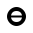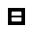sc_node_struct ScType::NodeStruct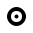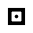sc_node_role_relation ScType::NodeRole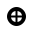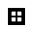sc_node_norole_relation ScType::NodeNoRole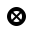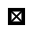sc_node_class ScType::NodeClass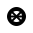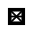sc_node_abstract ScType::NodeAbstract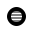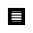sc_node_material ScType::NodeMaterial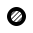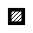There are old keynodes, that used for backward compatibility:

Keynode Equal sc-type New keynode
sc_arc_main ScType::EdgeAccessConstPosPerm sc_edge_main
sc_edge ScType::EdgeUCommon sc_edge_ucommon
sc_arc_common ScType::EdgeDCommon sc_edge_dcommon
sc_arc_access ScType::EdgeAccess sc_edge_access
sc_node_not_relation ScType::NodeClass sc_node_class
sc_node_not_binary_tuple ScType::NodeTuple sc_node_tuple

There is an example of usage:

SCs-code Equal SCg-code
```a <- sc_node_class;;
a _-> _b;;
_b <- sc_node_material;;
```
```_x => nrel_y: t;;
nrel_y <- sc_node_norole_relation;;
```